# Cypripedium candidum function-based MPMs

In this vignette, we will use the cypdata dataset to illustrate the estimation of function-based MPMs. To reduce file size, we have prevented some statements from running if they produce long stretches of output. Examples include most summary() calls - we include hashtagged versions of these calls, and our text assumes that the user runs these statements without hashtags. We have also written this vignette using the default Ehrlén format for all historical MPMs. All examples can be run in deVries format as well. This vignette is only a sample analysis. Detailed information and instructions on using lefko3 are available through a free online e-book called lefko3: a gentle introduction, available on the projects page of the Shefferson lab website. This website also includes links to long-format vignettes and other demonstrations of this package.

### ORGANISM AND POPULATION

The white lady’s slipper, Cypripedium candidum, is a North American perennial herb in the family Orchidaceae. It is long-lived and of conservation concern. The population from which the dataset originates is located within a state nature preserve located in northeastern Illinois, USA. The population was monitored annually from 2004 to 2009, with two monitoring sessions per year. Monitoring sessions took roughly 2 weeks each, and included complete censuses of the population divided into sections referred to as patches. Each monitoring session consisted of searches for previously recorded individuals, which were located according to coordinates relative to fixed stakes at the site, followed by a search for new individuals. Data recorded per individual included: the location, the number of non-flowering sprouts, the number of flowering sprouts, the number of flowers per flowering sprout, and the number of fruit pods per sprout (only in the second monitoring session per year, once fruiting had occurred). Location was used to infer individual identity. More information about this population and its characteristics is given in Shefferson et al. (2001) and Shefferson, Mizuta, and Hutchings (2017).

### BASIC WORKFLOW

Population matrix projection modeling requires an appropriate life history model showing how all stages and transitions are related. The figure below shows a very general life history model detailing these relationships in Cypripedium candidum. The first stage of life is the dormant seed, although a seed may germinate in the year following seed production, in which case the first stage of life is the protocorm. The protocorm is the first germinated stage - an underground, mycoheterotrophic stage unique to the families Orchidaceae and Pyrolaceae. There are three years of protocorm stages, followed by a seedling stage, and finally a set of stages that comprise the size-classified adult portion of life. The figure shows 49 such stages, each for a different number of stems (including zero for vegetative dormancy) and one of two reproductive statuses. These stages may be reorganized for different circumstances (more on this later).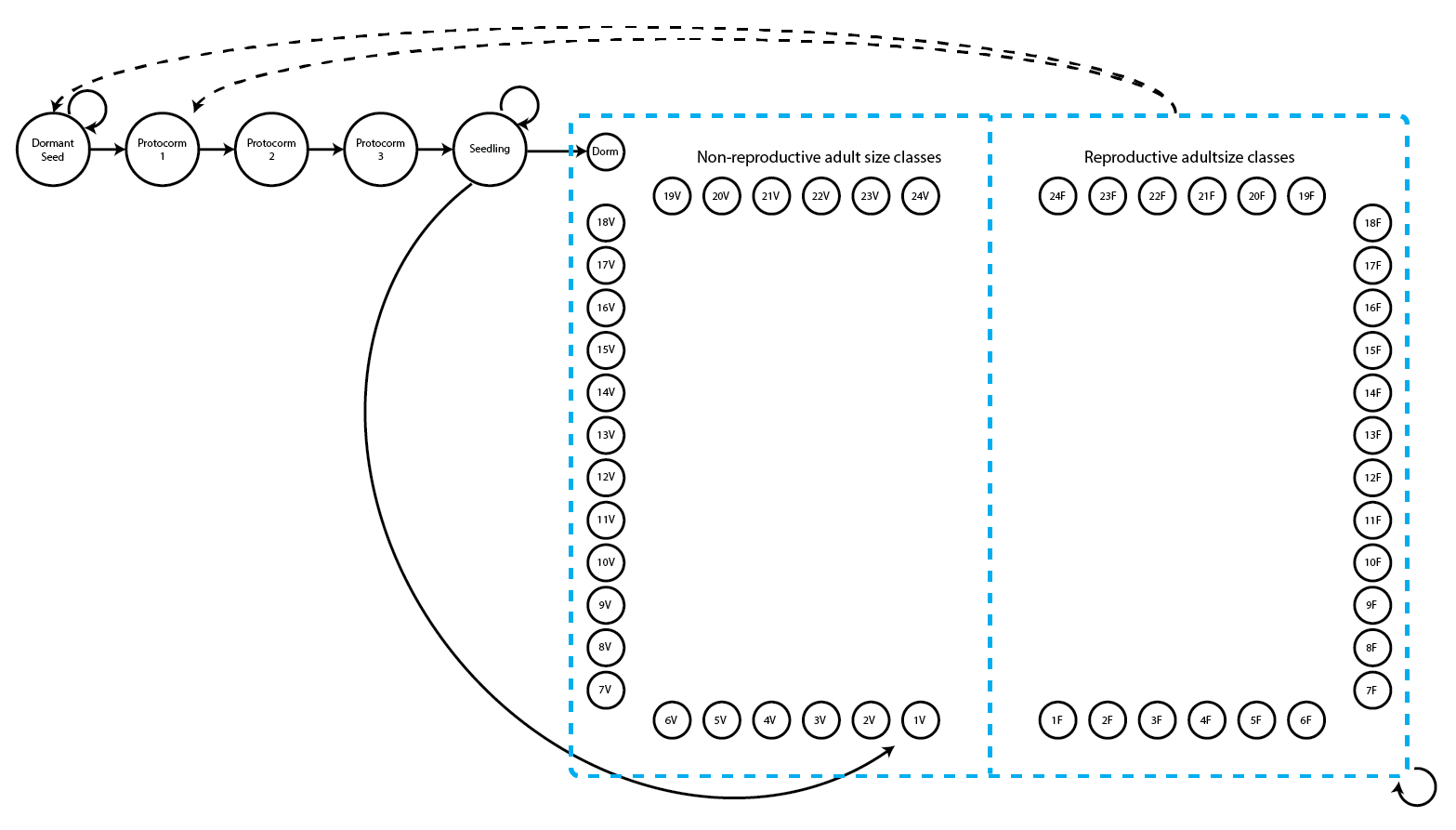Figure 5.1. Life history model of Cypripedium candidum. Survival transitions are indicated with solid arrows, while fecundity transitions are indicated with dashed arrows.

We can see a variety of transitions within this figure. The juvenile stages are limited to fairly simple, progressive transitions. New recruits may enter the population directly from germination of a seed produced the previous year, in which case they start in the protocorm 1 stage, or they may begin as dormant seed. Dormant seed may remain dormant, die, or germinate into the protocorm 1 stage. Protocorms exist for up to 3 years, yielding the protocorm 1, 2, and 3 stages, without any possibility of staying within each of these stages for more than a single year. Protocorm 3 leads to a seedling stage, in which the plant may persist for many years before becoming mature. Here, maturity does not really refer to reproduction per se, but rather to a morphology indistinguishable from a reproductive plant except for the lack of a flower. The first mature stage is usually either vegetative dormancy (Dorm), during which time the plant does not sprout, or a small, non-flowering adult (1V). Once in this portion of the life history, the plant may transition among 49 mature stages, including vegetative dormancy, 1-24 shoots without flowers, or 1-24 shoots with at least one flower (we have never observed a plant bigger than this in the population).

The horizontal dataset cypdata, and the ahistorical vertical dataset cypvert which is the same as cypdata but is structured differently, both include only data for the adult stages, and so later we will need to set juvenile transitions to constants from the literature. Let’s begin by clearing the memory, loading the package and the dataset, and looking over a summary of the latter.

rm(list=ls(all=TRUE))
library(lefko3)
data(cypdata)
#summary(cypdata)

#### Step 1. Life history model development

We will first describe the life history characterizing the population, matching it to our dataset with a stageframe for our Cypripedium candidum dataset. Since this analysis will be function-based and all sizes have reasonable representation, we will include all observed size classes here. Since size is count-based in the Cypripedium candidum case, we will use all numbers of stems that occurred, representing the life history diagram shown previously.

sizevector <- c(0, 0, 0, 0, 0, seq(from = 0, t = 24), seq(from = 1, to = 24))
stagevector <- c("SD", "P1", "P2", "P3", "SL", "D", "V1", "V2", "V3", "V4", "V5",
"V6", "V7", "V8", "V9", "V10", "V11", "V12", "V13", "V14", "V15", "V16", "V17",
"V18", "V19", "V20", "V21", "V22", "V23", "V24", "F1", "F2", "F3", "F4", "F5",
"F6", "F7", "F8", "F9", "F10", "F11", "F12", "F13", "F14", "F15", "F16", "F17",
"F18", "F19", "F20", "F21", "F22", "F23", "F24")
repvector <- c(0, 0, 0, 0, 0, rep(0, 25), rep(1, 24))
obsvector <- c(0, 0, 0, 0, 0, 0, rep(1, 48))
matvector <- c(0, 0, 0, 0, 0, rep(1, 49))
immvector <- c(0, 1, 1, 1, 1, rep(0, 49))
propvector <- c(1, rep(0, 53))
indataset <- c(0, 0, 0, 0, 0, rep(1, 49))
comments <- c("Dormant seed", "Yr1 protocorm", "Yr2 protocorm", "Yr3 protocorm",

cypframe <- sf_create(sizes = sizevector, stagenames = stagevector,
repstatus = repvector, obsstatus = obsvector, matstatus = matvector,
propstatus = propvector, immstatus = immvector, indataset = indataset,
#cypframe

A close look at the resulting object, cypframe, shows a data frame that includes the following information in order for each stage: the stage’s name; the associated primary, secondary, and tertiary sizes; the minimum and maximum ages associated with the stage; its reproductive status; its status as an observable stage; its status as a propagule stage; its status as an immature stage; its status as a mature stage; whether it occurs in the dataset; the half-width, minimum, maximum, centroid, and full width of its primary, secondary, and tertiary size class bins; stage group number; and a comments field describing the stage. We only used a primary size variable, so values for the secondary and tertiary sizes are NAs. Stage names and combinations of characteristics occurring within the dataset must be unique to prevent estimation errors, and the comments field may be edited to include any information deemed pertinent.

#### Step 2a. Data standardization

Now we will standardize our vertical dataset into a historically-formatted vertical (hfv) data frame using the verticalize3() function. The resulting dataset will have each individual’s observed life history broken up into states corresponding to three consecutive monitoring occasions (years) per row, with plant identity marked in each row.

vertdata <- verticalize3(data = cypdata, noyears = 6, firstyear = 2004,
patchidcol = "patch", individcol = "plantid", blocksize = 4,
sizeacol = "Inf2.04", sizebcol = "Inf.04", sizeccol = "Veg.04",
repstracol = "Inf.04", repstrbcol = "Inf2.04", fecacol = "Pod.04",
stageassign = cypframe, stagesize = "sizeadded", NAas0 = TRUE)
#summary(vertdata)

In the above code, we described the input dataset in a way that allows R to reorganize it appropriately. Note that in the input to the function, we utilized a repeating pattern of variable names arranged in the same order for each monitoring occasion. This arrangement allows us to enter only the first variable in each set, as long as noyears and blocksize are set properly and no gaps or shuffles appear among columns in the dataset. lefko3’s data management functions do not require such repeating patterns, but such patterns do make the required function input much more succinct. Without these patterns, column names should be input as vectors in the correct temporal order for each input option, and the blocksize option should be omitted.

The output dataset includes a number of summary variables, but the data is essentially broken down into groups of three consecutive monitoring occasions each (occasions t+1, t, and t-1, corresponding to year3, year2, and year1 in the output, respectively), with individuals spread across multiple rows. The output dataset is further limited to those entries in which the individual is alive in occasion t (year2), meaning that all rows in which an individual is dead or not yet recruited in occasion t are dropped. We end up with 320 rows of data and 54 variables.

This reorganized dataset includes a set of interesting terms that were automatically calculated: the sizeadded group of three variables. These are sums of the size variables for each occasion, such that size1added is calculated as sizea1 + sizeb1 + sizec1. This term may or may not make sense depending on the dataset. In this dataset, the full size of the individual in each occasion is this sum, because size is determined as the number of stems per plant, and the three original size columns give the numbers of three different kinds of stems: Veg, Inf, and Inf2 are the numbers of non-reproductive stems, single-flowered inflorescences, and double-flowered inflorescences, respectively, per plant per occasion (an inflorescence is a single flowering stem, and no inflorescence has more than two flowers). Since size is given as the total number of stems in this example, we will use the sizeadded group of variables to code individual size.

Size and fecundity are both count variables here, and we need to determine the appropriate distribution to use in both cases. Let’s look at histograms of size and fecundity in occasion t.

par(mfrow=c(1,2))
hist(vertdata$size2added, main = "Size in time t", xlab = "No. of stems in time t") hist(subset(vertdata, repstatus2 == 1)$feca2, main = "Fecundity in time t",
xlab = "No. of fruit pods in time t")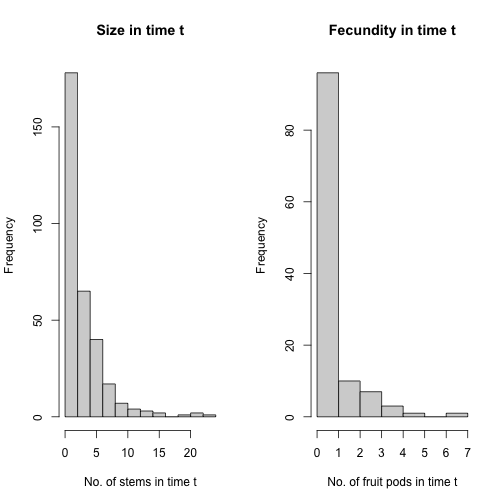Figure 5.2. Histogram of Cypripedium candidum size and fecundity in occasion t

Stem number has a very low mean, and the curve declines fairly smoothly. The Poisson or negative binomial distribution might work for size. Fecundity appears to have many zeros, suggesting that we might need a zero-inflated count distribution. Let’s conduct a formal set of tests to determine the appropriate distributions for size and fecundity. The size of zero is actually absorbed in an unobservable stage, vegetative dormancy (stage D), so we will not test for zero-inflation in the size variable. However, we will test for zero-inflation in fecundity, since fecundity is measured as the number of fruit pods produced, while all flowering individuals are treated as reproductive and so some individuals must have fecundity of zero.

sf_distrib(vertdata, sizea = c("size3added", "size2added"), obs3 = "obsstatus3",
fec = c("feca3", "feca2"), repst = c("repstatus3", "repstatus2"),
zisizea = FALSE)
#> Mean sizea is 3.653
#>
#> The variance in sizea is 13.41
#>
#> The probability of this dispersion level by chance assuming that
#> the true mean sizea = variance in sizea,
#> and an alternative hypothesis of overdispersion, is 3.721e-138
#>
#> Primary size is significantly overdispersed.
#>
#> Mean fec is 0.8036
#>
#> The variance in fec is 1.601
#>
#> The probability of this dispersion level by chance assuming that
#> the true mean fec = variance in fec,
#> and an alternative hypothesis of overdispersion, is 0.07088
#>
#> Dispersion level in fecundity matches expectation.
#>
#>
#> Mean lambda in fec is 0.4477
#> The actual number of 0s in fec is 65
#> The expected number of 0s in fec under the null hypothesis is 50.15
#> The probability of this deviation in 0s from expectation by chance is 1.742e-06
#>
#> Fecundity is significantly zero-inflated.

The results of these tests show us that size is overdispersed. Since we have no zeros in size, we will use the zero-truncated negative binomial distribution for this vital rate. Our tests of fecundity show no significant overdispersion, but the number of zeros exceeds expectation, suggesting that we use a zero-inflated Poisson distribution.

#### Step 2b. Vertical dataset standardization

We may also wish to see how to proceed if our original dataset is already in vertical, ahistorical format, or a format in which each row gives the state of an individual in a single monitoring occasion. This package also includes dataset cypvert, which is the same dataset as cypdata but set in ahistorical vertical format. Here, we use the historicalize3() function to deal with this dataset. First, let’s load the ahistorical vertical raw data file, look at its dimensions relative to the original, horizontal dataset, and finally view a summary.

data(cypvert)
dim(cypdata)
#>  77 29
dim(cypvert)
#>  322  14
#summary(cypvert)

This dataset has more rows and fewer columns, because we constructed the dataset by splitting the data for each individual across multiple rows. After three columns of identifying information (plantid, patch, and censor), a single column designates the time of occasion t, given as year2. This dataset then includes columns showing individual state in pairs of consecutive years corresponding to occasions t and t+1. State in occasion t-1 is not presented because this is an ahistorical dataset. This dataset includes the plantid variable, which is an individual identity term that allows us to associate rows with their individuals and so allows conversion. The historicalize3() function uses individual identity to reorganize datasets into historical vertical format.

vertdata2 <- historicalize3(data = cypvert, patchidcol = "patch",
individcol = "plantid", year2col = "year2", sizea2col = "Inf2.2",
sizea3col = "Inf2.3", sizeb2col = "Inf.2", sizeb3col = "Inf.3",
sizec2col = "Veg.2", sizec3col = "Veg.3", repstra2col = "Inf2.2",
repstra3col = "Inf2.3", repstrb2col = "Inf.2", repstrb3col = "Inf.3",
feca2col = "Pod.2", feca3col = "Pod.3", repstrrel = 2, stageassign = cypframe,
stagesize = "sizeadded", censorcol = "censor", censorkeep = 1, censor = FALSE,
NAas0 = TRUE, reduce = TRUE)
#summary(vertdata2)

We can compare the dimensions of the new vertical datasets. The lengths of the datasets are the same in terms of rows and columns, and the variables and data are the same although the order of the columns and rows might not match (see the summaries for comparison).

dim(vertdata)
#>  320  54
dim(vertdata2)
#>  320  54

One important consideration is the use of censor variables. Censoring a demographic dataset refers to the elimination of suspect data points from analysis. This is typically accomplished by including a binary variable in the dataset denoting whether an individual datum is to be kept or excluded. The objects vertdata and vertdata2 were both created without using censoring variables. However, because the datasets included censor variables (all data were set to be included, with no suspect data), we wished to incorporate those variables in the final datasets. Hence, although censor = FALSE in both the call to verticalize3() and the call to historicalize3(), we also noted censorcol = "censor" and censorkeep = 1 in the call to historicalize3() (the latter option specifies the value of the censor variable coding for a “good” datum). Failing to add these options to the call to historicalize3() will produce approximately the same dataset, but with some zeroes entering variables censor1, censor2, and censor3 in the historicalized dataset that do not exist in the first, verticalized dataset.

#### Step 2c. Provide supplemental information for matrix estimation

The next steps involve adding some external data to parameterize the matrices properly. We will use the supplemental() function to do so. The supplemental() function provides a means of inputting four kinds of data into MPM construction:

1. fixed transition values derived from other studies and added as constants to matrices;
2. proxy transition values when data for particular transitions do not exist and other, estimable transitions will be used as proxies;
3. reproductive multipliers to indicate which stages lead to the production of which stages, and at what level relative to estimated fecundity; and
4. survival multipliers, in cases in which proxy survival transitions are used but must be set at elevated or reduced levels relative to the original transition.

We will create two supplement tables, the first covering the historical analysis, and the second covering the ahistorical analysis. Each row refers to a specific transition, and in the historical case, there are codes for 23 given transitions (12 for the ahistorical case). The first nine of the historical transitions are set to specific probabilities (six for the ahistorical), and the next 12 are transitions that will be set to other, estimated transitions acting as proxies (four for the ahistorical; these are the non-NA transitions in eststage set below). The final two terms are fecundity multipliers. Based on the literature, the proxies for entry into the adult classes are transitions from dormancy, as below. Where necessary, we also use rep and mat as shorthand to code for all reproductive stages and all mature stages, respectively.

cypsupp3 <- supplemental(stage3 = c("SD", "SD", "P1", "P1", "P2", "P3", "SL",
"SL", "SL", "D", "V1", "V2", "V3", "D", "V1", "V2", "V3", "mat", "mat",
"mat", "mat", "SD", "P1"),
stage2 = c("SD", "SD", "SD", "SD", "P1", "P2", "P3", "SL", "SL", "SL", "SL",
"SL", "SL", "SL", "SL", "SL", "SL", "D", "V1", "V2", "V3", "rep", "rep"),
stage1 = c("SD", "rep", "SD", "rep", "SD", "P1", "P2", "P3", "SL", "P3", "P3",
"P3", "P3", "SL", "SL", "SL", "SL", "SL", "SL", "SL", "SL", "mat", "mat"),
eststage3 = c(NA, NA, NA, NA, NA, NA, NA, NA, NA, "D", "V1", "V2", "V3", "D",
"V1", "V2", "V3", "mat", "mat", "mat", "mat", NA, NA),
eststage2 = c(NA, NA, NA, NA, NA, NA, NA, NA, NA, "D", "D", "D", "D", "D",
"D", "D", "D", "D", "V1", "V2", "V3", NA, NA),
eststage1 = c(NA, NA, NA, NA, NA, NA, NA, NA, NA, "D", "D", "D", "D", "D",
"D", "D", "D", "V1", "V1", "V1", "V1", NA, NA),
givenrate = c(0.08, 0.08, 0.1, 0.1, 0.1, 0.1, 0.125, 0.2, 0.2, NA, NA, NA, NA,
NA, NA, NA, NA, NA, NA, NA, NA, NA, NA),
multiplier = c(NA, NA, NA, NA, NA, NA, NA, NA, NA, NA, NA, NA, NA, NA, NA, NA,
NA, 1, 1, 1, 1, 0.5, 0.5),
type = c("S", "S", "S", "S", "S", "S", "S", "S", "S", "S", "S", "S", "S", "S",
"S", "S", "S", "S", "S", "S", "S", "R", "R"),
type_t12 = c("S", "F", "S", "F", "S", "S", "S", "S", "S", "S", "S", "S", "S",
"S", "S", "S", "S", "S", "S", "S", "S", "S", "S"), stageframe = cypframe)

cypsupp2 <- supplemental(stage3 = c("SD", "P1", "P2", "P3", "SL", "SL", "D",
"V1", "V2", "V3", "SD", "P1"),
stage2 = c("SD", "SD", "P1", "P2", "P3", "SL", "SL", "SL", "SL", "SL", "rep",
"rep"),
eststage3 = c(NA, NA, NA, NA, NA, NA, "D", "V1", "V2", "V3", NA, NA),
eststage2 = c(NA, NA, NA, NA, NA, NA, "D", "D", "D", "D", NA, NA),
givenrate = c(0.08, 0.1, 0.1, 0.1, 0.125, 0.2, NA, NA, NA, NA, NA, NA),
multiplier = c(NA, NA, NA, NA, NA, NA, NA, NA, NA, NA, 0.5, 0.5),
type = c("S", "S", "S", "S", "S", "S", "S", "S", "S", "S", "R", "R"),
stageframe = cypframe, historical = FALSE)
#cypsupp3
#cypsupp2

These supplement tables provide the means to add external data to our MPMs by isolating specific transitions to be updated, and allow the use of shorthand to identify large groups of transitions for update (i.e. using mat, immat, rep, nrep, prop, npr, obs, nobs, groupX, or all to signify all mature stages, immature stages, reproductive stages, non-reproductive stages, propagule stages, non-propagule stages, observable stages, unobservable stages, stages within group X, or simply all stages, respectively). They also allow more powerful changes to MPMs, such as block reset of groups of transitions to zero or other constants.

#### Step 3. Tests of history, and vital rate modeling

Matrix estimation can proceed either via raw matrix methods, as initially outlined in Ehrlén (2000), or via function-based matrix development, essentially equivalent to the creation of complex integral projection models per Ellner and Rees (2006) and as further described in the non-Gaussian case in Shefferson, Warren II, and Pulliam (2014). In the raw MPM case, no vital rate models are estimated, whereas in function-based MPMs, vital rates are first analyzed via linear or non-linear models of the raw demographic dataset, and then functions are created that estimate these vital rates according the inputs given. Matrices are then estimated using these functions, as opposed to the raw data.

Prior to vital rate estimation, a number of key decisions need to be made regarding the assumptions underlying the vital rates, and their relationships with the factors under investigation. These decisions include the general modeling strategy, and the size and fecundity distributions.

#### Step 3a. General modeling strategy

Most function-based matrices, whether integral projection models or otherwise, are developed using either a generalized linear modeling (GLM) strategy, or a generalized linear mixed modeling (GLMM, or mixed) strategy. The former is more common and is simpler, but the latter is more theoretically sound because it provides a means of correcting the lack of independence inherent in datasets incorporating repeated sampling of the same individuals. The difference between the two methods with regards to vital rate modeling is strongly related to assumptions regarding individual and spatiotemporal variation in vital rates.

In both GLM and GLMM-based MPMs, the underlying dataset utilized is a vertical dataset. Each row of data gives the state of the individual in either two consecutive occasions (the ahistorical case), or three consecutive occasions (the historical case). Under the GLM framework, time in occasion t is a fixed categorical variable, and individual identity is ignored. Treating time as fixed implies that the actual monitoring occasions are the only times for which inference is sought. Thus, it would be inappropriate to infer future population dynamics after 2009 for a dataset collected between 2004 and 2009. Ignoring individual identity treats all transitions as independent, even though data originating from the same sampled individual is clearly not independent of that individual’s previous transitions. This may be interpreted as a form of pseudoreplication because strongly related data is used to estimate matrix elements and matrices that are assumed to be statistically independent of each other. This might impact demographic modeling by inflating Type 1 error in the linear modeling, yielding more significant terms in the chosen best-fit model and causing the retention of more terms than is warranted.

Under the GLMM (generalized linear mixed model, or mixed) framework, both time and individual identity can be treated as random categorical terms. This has two major implications. First, both time and individuals can be assumed to be random samples from a broader population of times and individuals for which inference is sought. Thus, sampled monitoring occasions represent a greater universe of years for which inference can be made. Second, treating individual as a random categorical term eliminates the pseudoreplication that is inherent in the GLM approach when individuals are monitored potentially many times, because each individual is assumed to be randomly drawn and associated with its own response distribution. Subpopulations may also be considered random, in which case they are assumed to have been sampled from all possible subpopulations whether or not other subpopulations actually exist. We encourage researchers to use the GLMM approach in their demographic work, but we have also included easy-to-use GLM functionality, since many will find the GLM approach particularly useful in cases where mixed modeling breaks down or takes too long.

#### Step 3b. Size and fecundity distributions

Once a general approach is decided upon, the next step is to choose the underlying distributions. The probabilities of survival, observation, and reproductive status are automatically set to the binomial distribution, and this cannot be altered. However, size transition probability and fecundity rate can be set to the Gaussian, Gamma, Poisson, or negative binomial distributions, with zero-inflated and zero-truncated versions of the Poisson and negative binomial also available. If size or fecundity rate is a continuous variable, then it should be set to the Gaussian distribution if not too right-skewed; otherwise, the Gamma distribution may be better (although the Gamma distribution requires only positive data). If size or fecundity is a count variable, then it should be set to the Poisson distribution if the mean equals the variance. The negative binomial distribution is provided in cases where the assumption that the mean equals the variance is clearly broken.

The Poisson and the negative binomial distributions both predict specific numbers of zeros in the response variable. If excess zeros occur within the dataset, then a zero-inflated Poisson or negative binomial distribution may be used. These modeling approaches work by parameterizing a binomial model, typically with a logit link, to predict zero responses. The Poisson or negative binomial is then used to predict non-zero responses. This model is really two separate models in which zeros are assumed to be predicted under potentially different processes than the remaining counts. Because an extra model is built to cover the occurrence of zeros, zero-inflated models are more complex and can include many more parameters than their non-inflated counterparts. The principle of parsimony suggests that they should only be used when there are significantly more zeros than expected.

Cases may arise in which zeros do not exist in either size or fecundity. For these situations, we provide zero-truncated distributions. This may occur in size if all cases of size = 0 are absorbed by observation status, leaving only positive integers for the size of observed individuals. For example, if an unobservable stage such as vegetative dormancy occurs and absorbs all cases of size = 0, then a zero-truncated Poisson or negative binomial distribution will be more appropriate than the equivalent distribution without zero-truncation. It can also occur if all cases of fecundity = 0 are absorbed by reproductive status.

#### Step 3c. Model building and selection

In lefko3, function modelsearch() is the workhorse that conducts vital rate model estimation. Here, we will create a full suite of vital rate models for the Cypripedium candidum dataset. Before proceeding, we need to decide on the linear model building strategy, the correct vital rates to model, the proper statistical distributions for estimated vital rates, the proper parameterization of each vital rate, and the strategy for determination of the best-fit model for each vital rate.

First, we must determine the model building strategy. In most cases, the best procedure will be through mixed linear models in which monitoring occasion and individual identity are random terms. We will set monitoring occasion as random because we wish to make inferences for the population as a whole and do not wish to restrict ourselves to inference only for the years monitored. We will set individual identity as random because many or most of the individuals that we have sampled to produce our dataset yield multiple observation data points across time. Thus, we will set approach = "mixed". To make sure that time and individual identity are treated as random, we will set the proper variable names for indiv and year, corresponding to individual identity (individ by default), and to occasion t (year2 by default). The year.as.random option is set to random by default, and leaving it this way also means that R will randomly draw coefficient values for years with inestimable coefficients. Setting year.as.random to FALSE would make time a fixed categorical variable.

Next, we must determine which vital rates to model. Function modelsearch() estimates up to fourteen vital rate models:

1. survival probability from occasion t to occasion t+1,

2. observation probability in occasion t+1 assuming survival until that time,

3. primary size in occasion t+1 assuming survival and observation in that time,

4. secondary size in occasion t+1 assuming survival and observation in that time,

5. tertiary size in occasion t+1 assuming survival and observation in that time,

6. reproduction status in occasion t+1 assuming survival and observation until that time,

7. fecundity rate assuming survival until and observation and reproduction in the occasion of production of offspring (occasion t or t+1; mature only),

8. juvenile survival probability from occasion t to occasion t+1,

9. juvenile observation probability in occasion t+1 assuming survival until that time,

10. juvenile primary size in occasion t+1 assuming survival and observation in that time,

11. juvenile secondary size in occasion t+1 assuming survival and observation in that time,

12. juvenile tertiary size in occasion t+1 assuming survival and observation in that time,

13. reproduction status in occasion t+1 assuming survival and observation until that time of a juvenile in occasion t that is becoming mature in occasion t+1, and

14. maturity status in occasion t+1 assuming survival until that time of a juvenile in occasion t.

The default settings for modelsearch estimate 1) survival probability, 3) primary size distribution, and 7) fecundity, which are the minimum three vital rates required for a full MPM. Observation probability (option obs in vitalrates) should only be included when a life history stage or size exists that cannot be observed. For example, in the case of a plant with vegetative dormancy, the observation probability can be thought of as the sprouting probability, which is a biologically meaningful vital rate (Shefferson et al. 2001). Reproduction status (option repst in vitalrates) should only be modeled if size classification needs to be stratified by the ability to reproduce, as when equivalently sized stages exist differing in the ability to reproduce. Secondary and tertiary size should only be modeled when two or three size variables are used in stage classification. Since Cypripedium candidum is capable of long bouts of vegetative dormancy, since we wish to stratify the population into reproductive and non-reproductive adults of the same size classes, and since we have no data derived from juvenile individuals, we will set vitalrates = c("surv", "obs", "size", "repst", "fec") and leave the juvenile-related options at their defaults.

Third, we need to set the proper statistical distribution for each parameter. Survival probability, observation probability, and reproductive status (and maturity status) are all modeled as binomial variables, and this cannot be changed. Size was measured as the number of stems and so is a count variable. Likewise, fecundity is actually estimated as the number of fruits produced per plant, and so is also a count variable. We have already performed tests for overdispersion and zero-inflation, and we are also aware that size in observed stages cannot equal 0, requiring zero truncation in that parameter. So we will set size to the zero-truncated negative binomial distribution, and fecundity to the zero-inflated Poisson distribution.

Fourth, we need the proper model parameterizations for each vital rate, using the suite option. The default, suite = "main", under the mixed model setting (approach = "mixed") starts with the estimation of global models that include size and reproductive status in occasions t and t-1 as fixed factors, with individual identity and time in occasion t (year t) set as random categorical terms. Other terms can be specified, including individual covariates, spatial density, and age. Setting suite = "full" will yield global models that also include all two-way interactions. The default under the GLM setting (approach = "glm") makes time in occasion t a fixed term and drops individual identity from consideration. The global model under suite = "full" then includes all fixed factors noted before, plus all two-way interactions between fixed factors ("full" is the only setting with interaction terms). If the population is not stratified by reproductive status, then suite = "size" will eliminate reproductive status terms and use all others in the global model. If size is not important, then suite = "rep" will eliminate size but keep reproductive status and all other terms. Finally, suite = "cons" will result in a global model in which neither reproductive status nor size are considered, although optional terms such as age or spatial density may be still be incorporated. We will set suite = "full".

Finally, we need to determine the proper strategy for the determination of the best-fit model. Model building proceeds through the dredge function in package MuMIn (Bartoń 2014), and each model has an associated AICc value. The default setting in lefko3 (bestfit = "AICc&k") will compare all models within 2.0 AICc units of the model with $$\Delta AICc = 0$$, and choose the one with the lowest degrees of freedom. This approach is generally better than the alternative, which simply uses the model with $$\Delta AICc = 0$$ (bestfit = "AICc"), as all models within 2.0 AICc units of that model are equally parsimonious and so fewer degrees of freedom result from fewer parameters estimated (Burnham and Anderson 2002).

cypmodels3 <- modelsearch(vertdata, historical = TRUE, approach = "mixed",
vitalrates = c("surv", "obs", "size", "repst", "fec"), sizedist = "negbin",
size.trunc = TRUE, fecdist = "poisson", fec.zero = TRUE, suite = "full",
#summary(cypmodels3)

As modelsearch works, it produces text describing its current processes and any issues encountered. It is quite likely that many warning messages will appear, and these may be of use in understanding the dataset and how well it conforms to analytical assumptions. We have silenced most of this with the quiet = TRUE option, although some red text still indicates a few warnings that have slipped through. These are not to be terribly concerned about. However, we encourage users to allow the function to run unsilenced in case of severe modeling problems. Please read the documentation for functions lm() (stats package), glm() (stats package), glm.nb() (MASS package), lmer() (lme4 package), glmer() (lme4 package), glmmTMB() (glmmTMB package), zeroinfl() (pscl package), vglm() (VGAM package), and dredge() (MuMIn package) for further information on the sources of problems in such models.

In the output, we see historical terms determining primary size, suggesting that we cannot ignore history. As such, the models created above are actually only usable for the construction of historical models. We can also see that although the accuracy of our survival and observation status models is very high (94.7% and 95% respectively), the accuracy of our reproductive status model is only fair (71.5%), and the pseudo R2 for fecundity is only 32%. For comparison, we may wish to estimate ahistorical models. In that case, we also need linear models in which the global models tested do not include state at occasion t-1, as below.

cypmodels2 <- modelsearch(vertdata, historical = FALSE, approach = "mixed",
vitalrates = c("surv", "obs", "size", "repst", "fec"), sizedist = "negbin",
size.trunc = TRUE, fecdist = "poisson", fec.zero = TRUE, suite = "full",
#summary(cypmodels2)

Fewer models were estimated per dredge, since fewer parameters were tested in the global models (size and reproductive status in occasion t-1 were not included). So, the best-fit models should look a little bit different. However, a more thorough comparison will show that many of the best-fit models are similar between historical and ahistorical analysis, and that the only model different is the primary size model, which now shows only a y-intercept and random terms for monitoring occasion and individual. This is not guaranteed - in this case, it may be that the relatively small number of years and small overall sample size leaves too little power to find an impact of historical status on most vital rates. However, with size impacted by history, the choice of a historical model is better here.

#### Step 4. MPM estimation

Now we will create function-based MPMs. Let’s first create a set of ahistorical matrices.

cypmatrix2 <- flefko2(stageframe = cypframe, supplement = cypsupp2,
modelsuite = cypmodels2, data = vertdata)
#summary(cypmatrix2)

A quick glance at the summary output will highlight that many more elements are estimated for function-based matrices than for raw matrices - here, 2,459 out of 2,916 total elements (84.3%). In raw matrices, elements associated with transitions from specific stages are only estimated when individuals actually exist within those particular stages, and so the number of stages is strongly limited by the size of the dataset. In function-based matrices, in contrast, the linear models estimated allow the estimation of all elements that are theoretically possible (i.e. only structural zeros are not estimated), and so stages may be developed for the full range of sizes experienced by individuals.

The survival probability summaries at the bottom of the output provide a useful quality control check. These are calculated as the column sums from the associated survival-transition matrices. The use of externally-supplied rates and probabilities, as supplied via the supplement and overwrite tables, can result in some stages or stage-pairs having survival probabilities greater than 1.0, and if that occurs, then the supplement/overwrite tables need to be corrected to reduce survival to something reasonable. Our matrices here look fine.

The output of the flefko2() call includes several key elements. One of the most important is a data frame showing the order of matrices produced. Let’s take a look at this object.

cypmatrix2$labels #> pop patch year2 #> 1 1 1 2004 #> 2 1 1 2005 #> 3 1 1 2006 #> 4 1 1 2007 #> 5 1 1 2008 Here we see that our five matrices are annual matrices corresponding to the same population/patch. Other elements in this object include summaries of the stages used, in the order that they occur in the matrix. Since this is an ahistorical MPM, the key element here is cypmatrix2$ahstages. If this were a historical MPM, then cypmatrix2$ahstages would give the order of stages, and cypmatrix2$hstages would include the order of stage-pairs corresponding to the rows and columns in such MPMs.

Next, we will create a set of historical Lefkovitch matrices.

cypmatrix3 <- flefko3(stageframe = cypframe, supplement = cypsupp3,
modelsuite = cypmodels3, data = vertdata)
#summary(cypmatrix3)

We see many more total elements per matrix (over 8.5 million, in contrast to 2,916 in the ahistorical case), and many more rows and columns (54 rows and columns in the ahistorical case, and 542 = 2,916 rows and columns in the historical case). However, historical matrices are composed primarily of structural zeros. Thus, with only 117,908 + 2,352 = 120,260 elements estimated per matrix, only 1.4% of elements are non-zero (the equivalent percentage for the ahistorical case is 84.3%).

Now let’s estimate some element-wise mean matrices. Here, we will create the ahistorical and historical mean MPMs and look at their summaries.

tmeans2r <- lmean(cypmatrix2)
tmeans3r <- lmean(cypmatrix3)
#summary(tmeans2r)
#summary(tmeans3r)

It might help to do a more holistic comparison of the ahistorical vs. historical MPMs. Let’s create matrix plots of the ahistorical and historical means. For this purpose, we will use the image3() function. Let’s first view the ahistorical matrix image.

image3(tmeans2r, used = 1)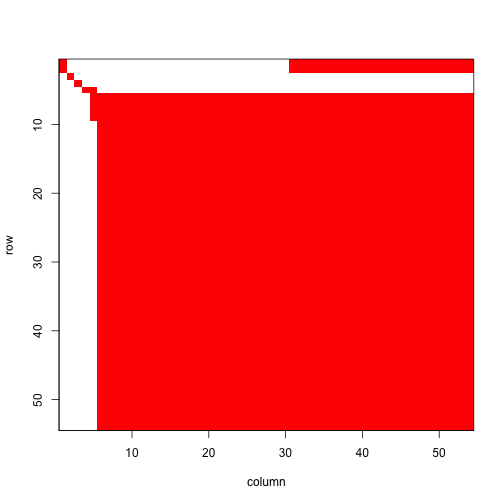Figure 5.3. Image of ahistorical element-wise matrix mean

#> []
#> NULL

This is a fairly large matrix, and rather dense though with obviously large clusters of zero elements particularly at the edges. Now let’s see the historical matrix.

image3(tmeans3r, used = 1)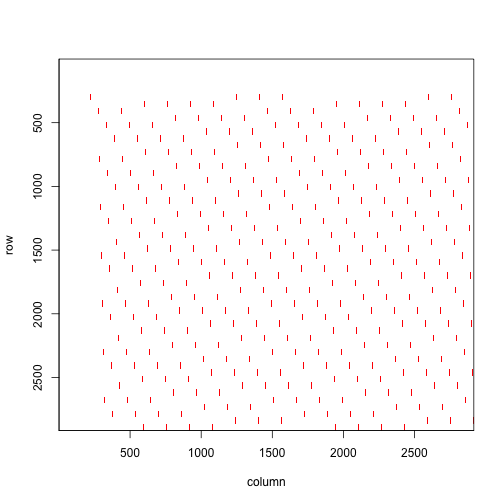Figure 5.4. Images of historical element-wise matrix mean

#> []
#> NULL

Historical matrices are often huge and always sparse, just as our historical matrix is in the plot above. Although there are roughly 8.5 million elements, very few are non-zero and those are identified as the red streaks in the matrix image above.

For further comparison, let’s decompose the mean historical matrix into conditional historical matrices. We’ll take a look at the matrix conditional on vegetative dormancy in occasion t-1.

condm3r <- cond_hmpm(tmeans3r)
#print(condm3r$Mcond[]$D, digits = 3)

This conditional matrix essentially takes the logically possible elements in which stage in time t-1 is D (vegetative dormancy), and creates a matrix with the dimensions and row and column order of an ahistorical matrix with them. Since vegetative dormancy is an adult stage, we do not see survival transitions from juvenile stages - only adult portions of the matrix are shown, including both survival and fecundity transitions.

#### Step 5. MPM analysis

Let’s now conduct some projection analyses. First, we will estimate the deterministic and stochastic population growth rates for these means. The set.seed() function makes our stochastic results reproducible.

set.seed(42)
cypann2s <- slambda3(cypmatrix2)
set.seed(42)
cypann3s <- slambda3(cypmatrix3)

lambda3(tmeans2r)
#>   pop patch    lambda
#> 1   1     1 0.9603441
lambda3(tmeans3r)
#>   pop patch    lambda
#> 1   1     1 0.9609416
cypann2s
#>   pop patch           a         var         sd           se
#> 1   1     1 -0.04043409 0.002352962 0.04850734 0.0004850734
cypann3s
#>   pop patch          a         var         sd           se
#> 1   1     1 -0.0397941 0.002286954 0.04782211 0.0004782211

These $$\lambda$$ values are similar to one another, as are estimates of $$a = \text{log} \lambda _{S}$$. Let’s take a look at a plot of annual deterministic growth rates (stochastic growth rate is calculated across time so is not included).

cypann3 <- lambda3(cypmatrix3)
cypann2 <- lambda3(cypmatrix2)

plot(lambda ~ year2, data = cypann2, xlab = "Year", ylab = "Lambda",
ylim = c(0.93, 0.98), type = "l", lty= 1, lwd = 2, bty = "n")
lines(lambda ~ year2, data = cypann3, lty = 2, lwd= 2, col = "red")
legend("bottomright", c("ahistorical", "historical"), lty = c(1, 2),
col = c("black", "red"), lwd = 2, bty = "n")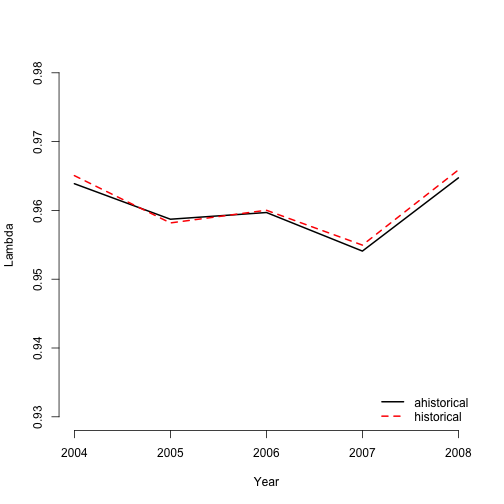Figure 5.5. Ahistorical vs. historical annual population growth rate

We find that annual $$\lambda$$ estimates differ only slightly between ahistorical and historical analyses.

Now let’s take a look at the stable stage distributions. We will look at deterministic and stochastic versions of the ahistorical and historical MPMs.

tm2ss <- stablestage3(tmeans2r)
tm3ss <- stablestage3(tmeans3r)
tm2ss_s <- stablestage3(cypmatrix2, stochastic = TRUE, seed = 42)
tm3ss_s <- stablestage3(cypmatrix3, stochastic = TRUE, seed = 42)

ss_put_together <- cbind.data.frame(tm2ss$ss_prop, tm3ss$ahist$ss_prop, tm2ss_s$ss_prop, tm3ss_s$ahist$ss_prop)
names(ss_put_together) <- c("det ahist", "det hist", "sto ahist", "sto hist")
rownames(ss_put_together) <- tm2ss$stage_id barplot(t(ss_put_together), beside=T, ylab = "Proportion", xlab = "Stage", ylim = c(0, 0.15), col = c("black", "orangered", "grey", "darkred"), bty = "n") legend("topright", c("det ahist", "det hist", "sto ahist", "sto hist"), col = c("black", "orangered", "grey", "darkred"), pch = 15, bty = "n")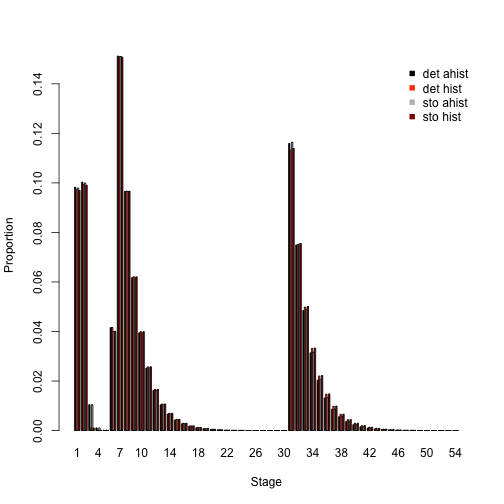Figure 5.6. Ahistorical vs. historically-corrected stable and long-run mean stage distribution Overall, these are very similar patterns. Whether ahistorical or historical, deterministic or stochastic, our analyses suggest that small non-reproductive adults take up the greatest share of the stable stage structure, with small reproductive adults coming next, and then dormant seed and 1st-year protocorms. Next let’s look at the reproductive values associated with both ahistorical and historical approaches. We will standardize against the maximum value in each case to make these comparable in a single plot (normally we would not standardize across analyses like this, using the first or minimum reproductive value in each case for standardization instead). tm2rv <- repvalue3(tmeans2r) tm3rv <- repvalue3(tmeans3r) tm2rv_s <- repvalue3(cypmatrix2, stochastic = TRUE, seed = 42) tm3rv_s <- repvalue3(cypmatrix3, stochastic = TRUE, seed = 42) rv_put_together <- cbind.data.frame((tm2rv$rep_value / max(tm2rv$rep_value)), (tm3rv$ahist$rep_value / max(tm3rv$ahist$rep_value)), (tm2rv_s$rep_value / max(tm2rv_s$rep_value)), (tm3rv_s$ahist$rep_value / max(tm3rv_s$ahist$rep_value))) names(rv_put_together) <- c("det ahist", "det hist", "sto ahist", "sto hist") rownames(rv_put_together) <- tm2rv$stage_id

barplot(t(rv_put_together), beside=T, ylab = "Relative rep value", xlab = "Stage",
ylim = c(0, 1.3), col = c("black", "orangered", "grey", "darkred"), bty = "n")
legend("topleft", c("det ahist", "det hist", "sto ahist", "sto hist"),
col = c("black", "orangered", "grey", "darkred"), pch = 15, bty = "n")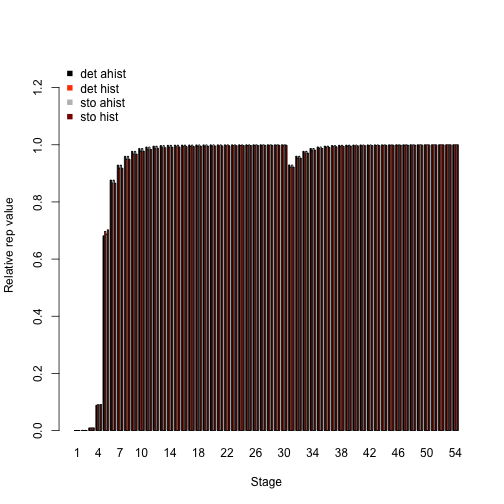Figure 5.7. Ahistorical vs. historically-corrected deterministic and stochastic reproductive values

We see roughly equivalent patterns here. All analyses show the smallest reproductive value in the juvenile stages, while adults have the greatest value, particularly medium- and large-sized adults of both flowering and non-flowering kinds. Interesting results in need of further study!

Let’s now conduct a sensitivity analysis, again using both deterministic and stochastic approaches. First we will look at the ahistorical MPM.

tm2sens <- sensitivity3(tmeans2r)
set.seed(42)
tm2sens_s <- sensitivity3(cypmatrix2, stochastic = TRUE)

writeLines(paste0("\nThe highest deterministic sensitivity value: ",
max(tm2sens$ah_sensmats[][which(tmeans2r$A[] > 0)])))
#>
#> The highest deterministic sensitivity value: 0.200249793902156
writeLines(paste0("This value is associated with element: ",
which(tm2sens$ah_sensmats[] == max(tm2sens$ah_sensmats[][which(tmeans2r$A[] > 0)])))) #> This value is associated with element: 378 writeLines(paste0("\nThe highest stochastic sensitivity value: ", max(tm2sens_s$ah_sensmats[][which(tmeans2r$A[] > 0)]))) #> #> The highest stochastic sensitivity value: 0.207109655095675 writeLines(paste0("This value is associated with element: ", which(tm2sens_s$ah_sensmats[] ==
max(tm2sens_s$ah_sensmats[][which(tmeans2r$A[] > 0)]))))
#> This value is associated with element: 378

The highest sensitivity value in both cases appears to be associated with the transition from 1-sprouted non-flowering adult to the largest flowering adult (element 378, in the 7th column and 54th row). Inspecting each sensitivity matrix also shows that transitions near that element in both matrices are also associated with rather high sensitivities.

Now let’s compare with the historical case. We will compare both the full historical sensitivity matrices, and also look at the historically-corrected deterministic sensitivities.

tm3sens <- sensitivity3(tmeans3r)
set.seed(42)
tm3sens_s <- sensitivity3(cypmatrix3, stochastic = TRUE)

writeLines(paste0("The highest deterministic sensitivity value: ",
max(tm3sens$h_sensmats[][which(tmeans3r$A[] > 0)])))
#> The highest deterministic sensitivity value: 0.0507110864421899
writeLines(paste0("This value is associated with element: ",
which(tm3sens$h_sensmats[] == max(tm3sens$h_sensmats[][which(tmeans3r$A[] > 0)])))) #> This value is associated with element: 962658 writeLines(paste0("The highest stochastic sensitivity value: ", max(tm3sens_s$h_sensmats[][which(tmeans3r$A[] > 0)]))) #> The highest stochastic sensitivity value: 0.0521953997830354 writeLines(paste0("This value is associated with element: ", which(tm3sens_s$h_sensmats[] ==
max(tm3sens_s$h_sensmats[][which(tmeans3r$A[] > 0)]))))
#> This value is associated with element: 962658

writeLines(paste0("The highest deterministic sensitivity value (historically-corrected): ",
max(tm3sens$ah_sensmats[][which(tmeans2r$A[] > 0)])))
#> The highest deterministic sensitivity value (historically-corrected): 0.198337403966719
writeLines(paste0("This value is associated with element: ",
which(tm3sens$ah_sensmats[] == max(tm3sens$ah_sensmats[][which(tmeans2r$A[] > 0)])))) #> This value is associated with element: 378 writeLines(paste0("The highest stochastic sensitivity value (historically-corrected): ", max(tm3sens_s$ah_sensmats[][which(tmeans2r$A[] > 0)]))) #> The highest stochastic sensitivity value (historically-corrected): 0.205686867202455 writeLines(paste0("This value is associated with element: ", which(tm3sens_s$ah_sensmats[] ==
max(tm3sens_s$ah_sensmats[][which(tmeans2r$A[] > 0)]))))
#> This value is associated with element: 378

We see both deterministic and stochastic sensitivity analyses supporting the transition from 1-sprouted non-flowering adult in occasions t-1 and t to the largest flowering adult in occasion t+1 (we can see this by dividing 962,658 by the number of stage pairs, which is 2916 - rounding up the answer gives us the matrix column , and the remainder from this division problem gives us the row ; the row and column numbers can then be checked against the hstages portion of the historical lefkoMat object used as input). The highest historically-corrected deterministic and stochastic sensitivity value is associated with the transition from 1-sprouted non-flowering adult to the largest flowering adult stage (element 378). Thus, our results reinforce the results of the ahistorical case.

Let’s now assess the elasticity of $$\lambda$$ to matrix elements, comparing the ahistorical to the historically-corrected case in both deterministic and stochastic analyses.

tm2elas <- elasticity3(tmeans2r)
tm3elas <- elasticity3(tmeans3r)
set.seed(42)
tm2elas_s <- elasticity3(cypmatrix2, stochastic = TRUE)
set.seed(42)
tm3elas_s <- elasticity3(cypmatrix3, stochastic = TRUE)

writeLines(paste0("The largest ahistorical deterministic elasticity is associated with element: ",
which(tm2elas$ah_elasmats[] == max(tm2elas$ah_elasmats[]))))
#> The largest ahistorical deterministic elasticity is associated with element: 331
writeLines(paste0("The largest historically-corrected deterministic elasticity is associated with element: ",
which(tm3elas$ah_elasmats[] == max(tm3elas$ah_elasmats[]))))
#> The largest historically-corrected deterministic elasticity is associated with element: 331
writeLines(paste0("The largest historical deterministic elasticity is associated with element: ",
which(tm3elas$h_elasmats[] == max(tm3elas$h_elasmats[]))))
#> The largest historical deterministic elasticity is associated with element: 962611

writeLines(paste0("The largest ahistorical stochastic elasticity is associated with element: ",
which(tm2elas_s$ah_elasmats[] == max(tm2elas_s$ah_elasmats[]))))
#> The largest ahistorical stochastic elasticity is associated with element: 331
writeLines(paste0("The largest historically-corrected stochastic elasticity is associated with element: ",
which(tm3elas_s$ah_elasmats[] == max(tm3elas_s$ah_elasmats[]))))
#> The largest historically-corrected stochastic elasticity is associated with element: 331
writeLines(paste0("The largest historical stochastic elasticity is associated with element: ",
which(tm3elas_s$h_elasmats[] == max(tm3elas_s$h_elasmats[]))))
#> The largest historical stochastic elasticity is associated with element: 962611

Ahistorical and historical, deterministic and stochastic analyses suggest that population growth rate is most elastic in response to stasis as 1-sprouted non-flowering adult (element 331). Indeed, element 962,611 in the historical case is actually stasis in this stage across times t-1, t, and t+1 (column: round(962611/2916) = 331; row: 962611 %% 2916 = 331). This is a remarkably consistent result, reinforcing the importance of this stage as seen in sensitivity analyses.

Now let’s compare the elasticity of population growth rate in relation to the core life history stages, via a barplot comparison.

elas_put_together <- cbind.data.frame(colSums(tm2elas$ah_elasmats[]), colSums(tm3elas$ah_elasmats[]), colSums(tm2elas_s$ah_elasmats[]), colSums(tm3elas_s$ah_elasmats[]))
names(elas_put_together) <- c("det ahist", "det hist", "sto ahist", "sto hist")
rownames(elas_put_together) <- tm2elas$ah_stages$stage_id

barplot(t(elas_put_together), beside=T, ylab = "Elasticity", xlab = "Stage",
col = c("black", "orangered", "grey", "darkred"), bty = "n")
legend("topright", c("det ahist", "det hist", "sto ahist", "sto hist"),
col = c("black", "orangered", "grey", "darkred"), pch = 15, bty = "n")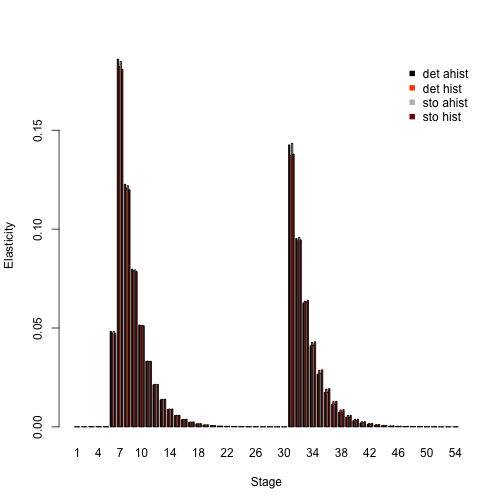Figure 5.8. Ahistorical vs. historically-corrected deterministic and stochastic elasticity to stage

Elasticity analyses in these plots look very similar. Population growth rates are most elastic in response to changes in stage 7, which is the one-sprouted non-flowering adult stage. Small adult stages have higher elasticity than larger adults and juveniles. Most of the differences between analyses here appear to lie in elasticity of small non-flowering vs. flowering adults in the ahistorical vs. historical comparison.

Finally, let’s take a look at how the importance of different kinds of transitions changes, by looking at elasticity sums. The plot will show that population growth rates are most elastic in response to changes in growth and shrinkage, with virtually no influence of fecundity.

tm2elas_sums <- summary(tm2elas)
tm3elas_sums <- summary(tm3elas)
tm2elas_s_sums <- summary(tm2elas_s)
tm3elas_s_sums <- summary(tm3elas_s)

elas_sums_together <- cbind.data.frame(tm2elas_sums$ahist[,2], tm3elas_sums$ahist[,2], tm2elas_s_sums$ahist[,2], tm3elas_s_sums$ahist[,2])
names(elas_sums_together) <- c("det ahist", "det hist", "sto ahist", "sto hist")
rownames(elas_sums_together) <- tm2elas_sums$ahist$category

barplot(t(elas_sums_together), beside=T, ylab = "Elasticity", xlab = "Transition",
col = c("black", "orangered", "grey", "darkred"), bty = "n")
legend("topright", c("det ahist", "det hist", "sto ahist", "sto hist"),
col = c("black", "orangered", "grey", "darkred"), pch = 15, bty = "n")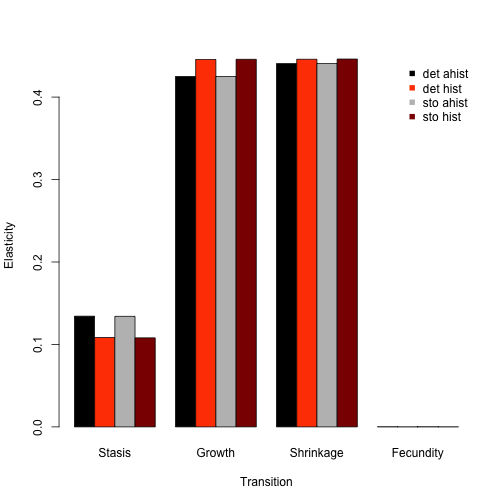Figure 5.9. Ahistorical vs. historically-corrected elasticity of lambda to transitions

Some users of lefko3 may wish to use conduct custom projections, perhaps involving density dependence or projected individual covariate inputs. Beginning with version 5.0, lefko3 includes function f_projection3(), which allows users to conduct projection simulations of function-based MPMs. This function takes the vital rate models that constitute the lefkoMod object input into matrix generating functions flefko3(), flefko2(), aflefko2(), and afleslie(), and builds matrices projecting the population forward according to whatever inputs the user has in mind. Function f_projection3() is different from function projection3() in that the latter requires a lefkoMat object as input, and uses the matrices in whatever projection the user has in mind. The former actually creates these matrices from scratch at each time step and so does not take matrices as inputs.

To illustrate how we might use this function, let’s run a conservation-focused analysis using the ahistorical vital rate models created above. We will ask what happens if we artificially pollinate these plants enough to double their fecundity. Let’s first create a lefkoDens object, which holds the density dependence information. Let’s also create an alternative supplement table with doubled fecundity.

c2d <- density_input(cypmatrix2, stage3 = c("SD", "P1", "SD", "P1"),
stage2 = c("SD", "SD", "rep", "rep"), style = 1,
time_delay = 1, alpha = 1, beta = 0, type = c(1, 1, 2, 2))
#c2d

cypsupp2alt <- supplemental(stage3 = c("SD", "P1", "P2", "P3", "SL", "SL", "D",
"V1", "V2", "V3", "SD", "P1"),
stage2 = c("SD", "SD", "P1", "P2", "P3", "SL", "SL", "SL", "SL", "SL", "rep",
"rep"),
eststage3 = c(NA, NA, NA, NA, NA, NA, "D", "V1", "V2", "V3", NA, NA),
eststage2 = c(NA, NA, NA, NA, NA, NA, "D", "D", "D", "D", NA, NA),
givenrate = c(0.08, 0.1, 0.1, 0.1, 0.125, 0.2, NA, NA, NA, NA, NA, NA),
multiplier = c(NA, NA, NA, NA, NA, NA, NA, NA, NA, NA, 0.5*2, 0.5*2),
type = c("S", "S", "S", "S", "S", "S", "S", "S", "S", "S", "R", "R"),
stageframe = cypframe, historical = FALSE)
#cypsupp2alt

Now let’s run two projections, the first using the original ahistorical information, and the second using the altered supplement table. We will run both as stochastic and density dependent, with ten replicates of each and 100 time steps.

set.seed(42)
basetrial <- f_projection3(format = 3, data = vertdata, supplement = cypsupp2,
stageframe = cypframe, modelsuite = cypmodels2, nreps = 10, times = 100,
stochastic = TRUE, growthonly = TRUE, substoch = 2, density = c2d, err_check = TRUE)
#> Warning: Option patch not set, so will set to first patch/population.
alttrial <- f_projection3(format = 3, data = vertdata, supplement = cypsupp2alt,
stageframe = cypframe, modelsuite = cypmodels2, nreps = 10, times = 100,
stochastic = TRUE, growthonly = TRUE, substoch = 2, density = c2d)
#> Warning: Option patch not set, so will set to first patch/population.

summary(basetrial)
#>
#> The input lefkoProj object covers 1 population-patches.
#> It includes 100 projected steps per replicate and 10 replicates.
#> The number of replicates with population size above the threshold size of 1 is as in
#> the following matrix, with pop-patches given by column and milepost times given by row:
#>     1 1
#> 1    10
#> 26   10
#> 51   10
#> 76   10
#> 101  10
summary(alttrial)
#>
#> The input lefkoProj object covers 1 population-patches.
#> It includes 100 projected steps per replicate and 10 replicates.
#> The number of replicates with population size above the threshold size of 1 is as in
#> the following matrix, with pop-patches given by column and milepost times given by row:
#>     1 1
#> 1    10
#> 26   10
#> 51   10
#> 76   10
#> 101  10

Let’s compare these two projections with plots.

par(mfrow = c(1,2))
plot(basetrial, ylim = c(0, 100))
plot(alttrial, ylim = c(0, 100))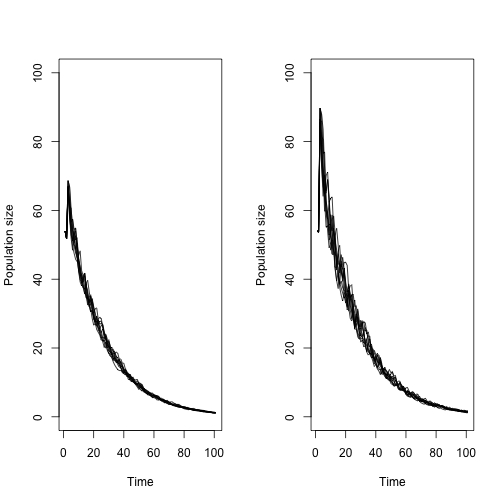Figure 5.10. Projections of Cypripedium population against boosted fecundity

Each plot shows 10 lines, one for each replicate. Both projections show decline, but the population appears to reach a higher high size early on under the boosted fecundity scenario. So, while boosting fecundity does not prevent extinction, it does seem to boost the population and probably lowers the overall chances of extinction within a short time window.

We have also created other literature to help users familiarize themselves with population analyses in general and using the package. Users should see our free e-manual called lefko3: a gentle introduction, as well as other vignettes including long-format and video vignettes, on the projects page of the Shefferson lab website.

## Acknowledgements

We are grateful to Joyce and George Proper, Ken Klick, and the many other volunteers who have helped amass the Cypripedium dataset, as well as to two anonymous reviewers whose scrutiny improved the quality of this vignette. The project resulting in this package and this tutorial was funded by Grant-In-Aid 19H03298 from the Japan Society for the Promotion of Science.

## Literature cited

Bartoń, Kamil A. 2014. MuMIn: Multi-Model Inference.” https://CRAN.R-project.org/package=MuMIn.
Burnham, Kenneth P., and David R. Anderson. 2002. Model Selection and Multimodel Inference: A Practical Information-Theoretic Approach. New York, New York, USA: Springer-Verlag New York, Inc.
Ehrlén, Johan. 2000. “The Dynamics of Plant Populations: Does the History of Individuals Matter?” Ecology 81 (6): 1675–84. https://doi.org/10.1890/0012-9658(2000)081[1675:TDOPPD]2.0.CO;2.
Ellner, Stephen P., and Mark Rees. 2006. “Integral Projection Models for Species with Complex Demography.” American Naturalist 167 (3): 410–28. https://doi.org/10.1086/499438.
Shefferson, Richard P., Ryo Mizuta, and Michael J. Hutchings. 2017. “Predicting Evolution in Response to Climate Change: The Example of Sprouting Probability in Three Dormancy-Prone Orchid Species.” Royal Society Open Science 4 (1): 160647. https://doi.org/10.1098/rsos.160647.
Shefferson, Richard P., Brett K. Sandercock, Joyce Proper, and Steven R. Beissinger. 2001. “Estimating Dormancy and Survival of a Rare Herbaceous Perennial Using Mark-Recapture Models.” Ecology 82 (1): 145–56. https://doi.org/10.1890/0012-9658(2001)082[0145:EDASOA]2.0.CO;2.
Shefferson, Richard P., Robert J. Warren II, and H. Ronald Pulliam. 2014. “Life History Costs Make Perfect Sprouting Maladaptive in Two Herbaceous Perennials.” Journal of Ecology 102 (5): 1318–28. https://doi.org/10.1111/1365-2745.12281.